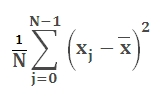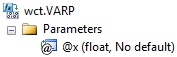# SQL Server population variance function

VARP

Updated: 28 February 2011

Note: This documentation is for the SQL2008 (and later) version of this XLeratorDB function, it is not compatible with SQL Server 2005.

Use VARP to return the variance for an entire population. This function differs from the built-in SQL Server VARP function in that it employs a two-pass and more numerically stable method for calculating the standard deviation and under certain conditions will return a different value than the built-in function.

For a population N having a distribution where the mean is not known, the population variance is defined as:SyntaxArguments
@x
the values used in the standard deviation calculation. @ x is an expression of type float or of a type that can be implicitly converted to float.
Return Types
float
Remarks
·         If you want measure the variance for a sample, then use the VAR function.
·         NULL values are not included in the standard deviation calculation.
·         STDEV is an aggregate function and follows the same conventions as all other aggregate functions in SQL Server.
·         If you have previously used the STDEV scalar function, the STDEV aggregate has a different syntax. The STDEV scalar function is no longer available in XLeratorDB/statistics2008, though you can still use the scalar STDEV _q.
Examples
SELECT wct.VARP(x) as VARP
FROM (VALUES
(91.3698),
(76.3382),
(74.5692),
(85.2957),
(99.0112),
(86.99),
(70.7837),
(72.834),
(78.1644),
(77.7472),
(66.0627),
(59.781),
(68.4793),
(78.6103),
(59.8621)
) n(x)

This produces the following result.
VARP
----------------------
113.557402750489

(1 row(s) affected)

In the following example, we will use demonstrate the difference between the SQL Server VARP calculation and XLeratorDB VARP calculation. Using the XLeratorDB/math SeriesFloat function to create a million rows of randomly generated values between 1,000,000,000 and 1,000,000,010
SELECT ROUND(SeriesValue * 10, 0) + 1000000000 as x
INTO #n
FROM wctMath.wct.SERIESFLOAT(0,1,'',1000000,'R')

SELECT VARP(x) as [SQL SERVER VARP]
,wct.VARP(x) as [XLDB VARP]
,VARP(x-1000000000) as [SQL SERVER VARP adjusted]
FROM #n

DROP TABLE #n

This produces the following result. Your results will vary, since the dataset is randomly generated. The first column shows the built-in SQL Server VARP calculation, the second shows the XLeratorDB calculation and the third column shows the built-in calculation for x minus 1,000,000,000.
SQL SERVER VARP              XLDB VARP SQL SERVER VARP adjusted
---------------------- ---------------------- ------------------------
250181.844992       8.49856400542397           8.498564005436

(1 row(s) affected)

### SupportCopyright 2008-2023 Westclintech LLC         Privacy Policy        Terms of Service